# NCERT solutions for Class 9 Maths chapter 7 - Triangles [Latest edition]

#### Chapters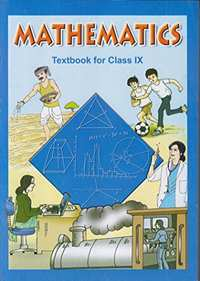## Solutions for Chapter 7: Triangles

Below listed, you can find solutions for Chapter 7 of CBSE NCERT for Class 9 Maths.

Exercise 7.1Exercise 7.2Exercise 7.3Exercise 7.4Exercise 7.5
Exercise 7.1 [Pages 118 - 120]

### NCERT solutions for Class 9 Maths Chapter 7 Triangles Exercise 7.1 [Pages 118 - 120]

Exercise 7.1 | Q 1 | Page 118

In quadrilateral ACBD, AC = AD and AB bisects ∠A (See the given figure). Show that ΔABC ≅ ΔABD. What can you say about BC and BD?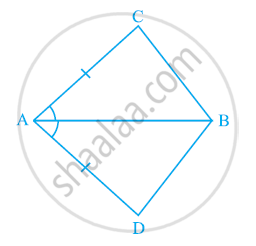Exercise 7.1 | Q 2 | Page 119

ABCD is a quadrilateral in which AD = BC and ∠DAB = ∠CBA (See the given figure). Prove that

(i) ΔABD ≅ ΔBAC

(ii) BD = AC

(iii) ∠ABD = ∠BAC.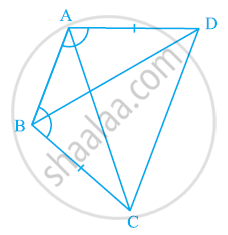Exercise 7.1 | Q 3 | Page 119

AD and BC are equal perpendiculars to a line segment AB (See the given figure). Show that CD bisects AB.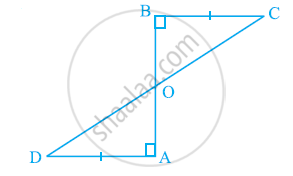Exercise 7.1 | Q 4 | Page 119

l and m are two parallel lines intersected by another pair of parallel lines p and q (see the given figure). Show that ΔABC ≅ ΔCDA.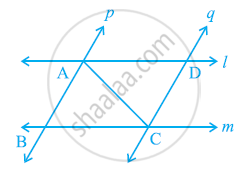Exercise 7.1 | Q 5 | Page 119

Line l is the bisector of an angle ∠A and B is any point on l. BP and BQ are perpendiculars from B to the arms of ∠A (see the given figure). Show that:-

(i) ΔAPB ≅ ΔAQB

(ii) BP = BQ or B is equidistant from the arms of ∠A.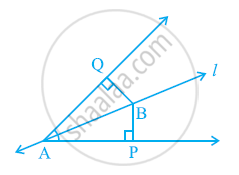Exercise 7.1 | Q 6 | Page 120

In the given figure, AC = AE, AB = AD and ∠BAD = ∠EAC. Show that BC = DE.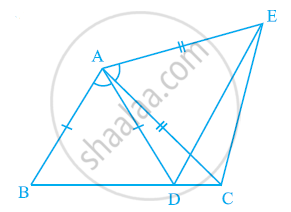Exercise 7.1 | Q 7 | Page 120

AB is a line segment and P is its mid-point. D and E are points on the same side of AB such that ∠BAD = ∠ABE and ∠EPA = ∠DPB (See the given figure). Show that

(i) ΔDAP ≅ ΔEBP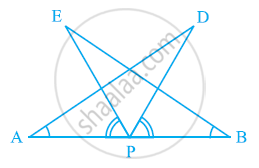Exercise 7.1 | Q 8 | Page 120

In right triangle ABC, right angled at C, M is the mid-point of hypotenuse AB. C is joined to M and produced to a point D such that DM = CM. Point D is joined to point B (see the given figure). Show that:

(i) ΔAMC ≅ ΔBMD

(ii) ∠DBC is a right angle.

(iii) ΔDBC ≅ ΔACB

(iv) CM = 1/2AB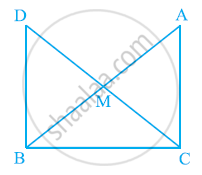Exercise 7.2 [Pages 123 - 124]

### NCERT solutions for Class 9 Maths Chapter 7 Triangles Exercise 7.2 [Pages 123 - 124]

Exercise 7.2 | Q 1 | Page 123

In an isosceles triangle ABC, with AB = AC, the bisectors of ∠B and ∠C intersect each other at O. Join A to O. Show that: (i) OB = OC (ii) AO bisects ∠A

Exercise 7.2 | Q 2 | Page 123

In ΔABC, AD is the perpendicular bisector of BC (see the given figure). Show that ΔABC is an isosceles triangle in which AB = AC.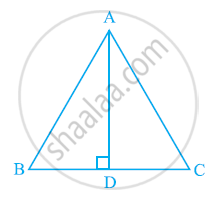Exercise 7.2 | Q 3 | Page 124

ABC is an isosceles triangle in which altitudes BE and CF are drawn to equal sides AC and AB respectively (see the given figure). Show that these altitudes are equal.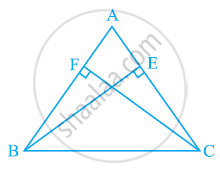Exercise 7.2 | Q 4 | Page 124

ABC is a triangle in which altitudes BE and CF to sides AC and AB are equal (see the given figure). Show that

(i) ΔABE ≅ ΔACF

(ii) AB = AC, i.e., ABC is an isosceles triangle.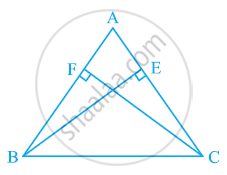Exercise 7.2 | Q 5 | Page 124

ABC and DBC are two isosceles triangles on the same base BC (see the given figure). Show that ∠ABD = ∠ACD.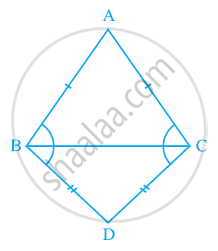Exercise 7.2 | Q 6 | Page 124

ΔABC is an isosceles triangle in which AB = AC. Side BA is produced to D such that AD = AB (see the given figure). Show that ∠BCD is a right angle.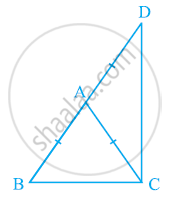Exercise 7.2 | Q 7 | Page 124

ABC is a right angled triangle in which ∠A = 90º and AB = AC. Find ∠B and ∠C.

Exercise 7.2 | Q 8 | Page 124

Show that the angles of an equilateral triangle are 60º each.

Exercise 7.3 [Page 128]

### NCERT solutions for Class 9 Maths Chapter 7 Triangles Exercise 7.3 [Page 128]

Exercise 7.3 | Q 1 | Page 128

ΔABC and ΔDBC are two isosceles triangles on the same base BC and vertices A and D are on the same side of BC (see the given figure). If AD is extended to intersect BC at P, show that

(i) ΔABD ≅ ΔACD

(ii) ΔABP ≅ ΔACP

(iii) AP bisects ∠A as well as ∠D.

(iv) AP is the perpendicular bisector of BC.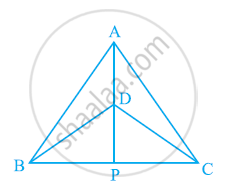Exercise 7.3 | Q 2 | Page 128

AD is an altitude of an isosceles triangles ABC in which AB = AC. Show that

Exercise 7.3 | Q 3 | Page 128

Two sides AB and BC and median AM of one triangle ABC are respectively equal to sides PQ and QR and median PN of ΔPQR (see the given figure). Show that:

(i) ΔABM ≅ ΔPQN

(ii) ΔABC ≅ ΔPQR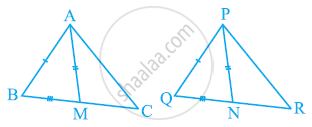Exercise 7.3 | Q 4 | Page 128

BE and CF are two equal altitudes of a triangle ABC. Using RHS congruence rule, prove that the triangle ABC is isosceles.

Exercise 7.3 | Q 5 | Page 128

ABC is an isosceles triangle with AB = AC. Drawn AP ⊥ BC to show that ∠B = ∠C.

Exercise 7.4 [Pages 132 - 133]

### NCERT solutions for Class 9 Maths Chapter 7 Triangles Exercise 7.4 [Pages 132 - 133]

Exercise 7.4 | Q 1 | Page 132

Show that in a right angled triangle, the hypotenuse is the longest side.

Exercise 7.4 | Q 2 | Page 132

In the given figure sides AB and AC of ΔABC are extended to points P and Q respectively. Also, ∠PBC < ∠QCB. Show that AC > AB.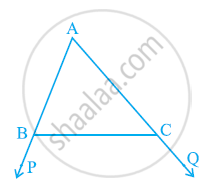Exercise 7.4 | Q 3 | Page 132

In the given figure, ∠B < ∠A and ∠C < ∠D. Show that AD < BC.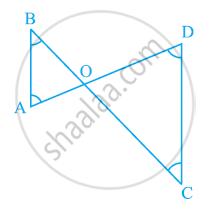Exercise 7.4 | Q 4 | Page 132

AB and CD are respectively the smallest and longest sides of a quadrilateral ABCD (see the given figure). Show that ∠A > ∠C and ∠B > ∠D.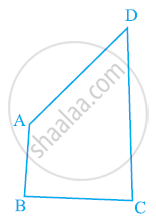Exercise 7.4 | Q 5 | Page 132

In the given figure, PR > PQ and PS bisects ∠QPR. Prove that ∠PSR >∠PSQ.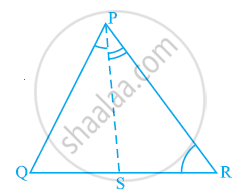Exercise 7.4 | Q 6 | Page 133

Show that of all line segments drawn from a given point not on it, the perpendicular line segment is the shortest.

Exercise 7.5 [Page 133]

### NCERT solutions for Class 9 Maths Chapter 7 Triangles Exercise 7.5 [Page 133]

Exercise 7.5 | Q 1 | Page 133

ABC is a triangle. Locate a point in the interior of ΔABC which is equidistant from all the vertices of ΔABC.

Exercise 7.5 | Q 2 | Page 133

In a triangle locate a point in its interior which is equidistant from all the sides of the triangle.

Exercise 7.5 | Q 3 | Page 133

In a huge park people are concentrated at three points (see the given figure):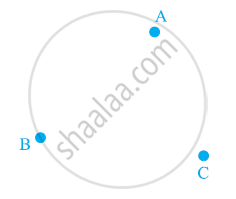A: where there are different slides and swings for children,

B: near which a man-made lake is situated,

C: which is near to a large parking and exit.

Where should an ice-cream parlour be set up so that maximum number of persons can approach it?

(Hint: The parlor should be equidistant from A, B and C)

Exercise 7.5 | Q 4 | Page 133

Complete the hexagonal and star shaped rangolies (see the given figures) by filling them with as many equilateral triangles of side 1 cm as you can. Count the number of triangles in each case. Which has more triangles?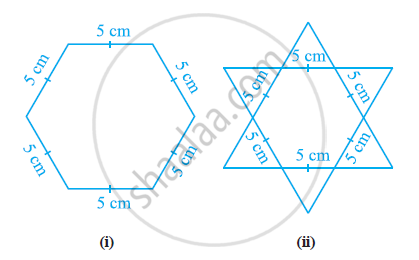## Solutions for Chapter 7: Triangles

Exercise 7.1Exercise 7.2Exercise 7.3Exercise 7.4Exercise 7.5## NCERT solutions for Class 9 Maths chapter 7 - Triangles

Shaalaa.com has the CBSE Mathematics Class 9 Maths CBSE solutions in a manner that help students grasp basic concepts better and faster. The detailed, step-by-step solutions will help you understand the concepts better and clarify any confusion. NCERT solutions for Mathematics Class 9 Maths CBSE 7 (Triangles) include all questions with answers and detailed explanations. This will clear students' doubts about questions and improve their application skills while preparing for board exams.

Further, we at Shaalaa.com provide such solutions so students can prepare for written exams. NCERT textbook solutions can be a core help for self-study and provide excellent self-help guidance for students.

Concepts covered in Class 9 Maths chapter 7 Triangles are Concept of Triangles - Sides, Angles, Vertices, Interior and Exterior of Triangle, Properties of a Triangle, Some More Criteria for Congruence of Triangles, Inequalities in a Triangle, Criteria for Congruence of Triangles, Congruence of Triangles.

Using NCERT Class 9 Maths solutions Triangles exercise by students is an easy way to prepare for the exams, as they involve solutions arranged chapter-wise and also page-wise. The questions involved in NCERT Solutions are essential questions that can be asked in the final exam. Maximum CBSE Class 9 Maths students prefer NCERT Textbook Solutions to score more in exams.

Get the free view of Chapter 7, Triangles Class 9 Maths additional questions for Mathematics Class 9 Maths CBSE, and you can use Shaalaa.com to keep it handy for your exam preparation.